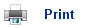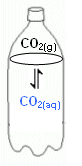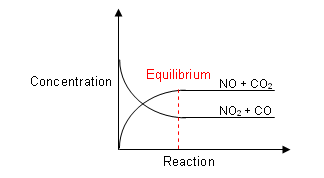# Equilibrium

• Equilibrium is a state of balance in which nothing changes.
• Chemical reactions are said to be in Dynamic Equilibrium when the amount of product and reactants remains the same and the forward and backward reactions are occuring at the same rate.
• Consider a bottle of soda water. The bottle and its contents make up a closed system.
• In this system, the CO2 is present in two states; a gas and as an aqueous solution in water.• If the bottle is left standing at a steady temperature for some time, the pressure of the carbon dioxide in the gas above the water will remain constant, and the concentration of carbon dioxide in the aqueous solution will remain constant.
• The system is at equilibrium.
• However, even though there is no overall change, there is still movement happening, this is why it is a Dynamic Equilibrium.
• Molecules of CO2 are leaving the water, while at the same time molecules are dissolving into it.

CO2(g)CO2(g)

• When the system is at equilibrium, the molecules enter and leave at the same rate.
• It is not only the carbon dioxide, but the water molecules themselves are also at dynamic equilibrium.
• H2 O molecules are constantly escaping into the gaseous phase, while at the same time molecules from the gas phase are condensing on the surface of the water.
• In a closed system (i.e. the closed bottle), molecules reach the point after a while where molecules are escaping and condensing at the same rate. The system is in dynamic equilibrium.

H2 O (aq)H2 O (g)

• The previous two examples of dynamic equilibrium were not chemical equilibrium, but rather physical processes involving liquid/gas change.
• A small proportion of the carbon dioxide within the water actually reacts with it. Hydrogencarbonate and hydrogen ions are formed:

CO2(aq) + H2 O (g)HCO3 -(aq) + H+(aq)

• On the molecular scale, CO2 molecules are constantly reacting with H2 O molecules to form HCO3 - and H+ ions; however at the same time the reverse is happening.
• It is said to be a reversible reaction.
• After an amount of time, the forward and backward reaction is occurring at the same rate, this is when the reaction is at dynamic equilibrium.
• When NO2 and CO are heated in a container they react together:

NO2(g) + CO (g)NO (g) + CO2(g)

• At the start of the reaction, no molecules of NO or CO2 are present, so there will be no reverse reaction.
• However, as the reaction continues, the products are made, and the reverse reaction can begin.
• It will occur slowly at first, but as the concentrations of NO and CO2 increase, the reverse reaction will occur at a faster rate.
• At the same time, the concentrations of NO2 and CO will be decreasing, so the forward reaction will be occurring at a slower rate.
• Eventually a point is reached where the forward rate is equal to the backward rate.
• At this point, the reactants are being converted into the products, at the same time as the products are being converted back into the reactants.
• It is at dynamic equilibrium, as both the forward and backward reactions are still occurring, but the concentrations of both products and reactants remain constant.## Position of Equilibrium

• There are many equilibrium mixtures possible for a given chemical reaction, depending on the concentrations of solutions, the pressure of gases and the temperature of the reaction.
• The term position of equilibrium can be used to describe a particular set of equilibrium concentrations for a reaction.
• If either the temperature, pressure or concentration of one of the substances changes, then the concentrations of all the substances will change until a new position of equilibrium is reached.
• Although the rates of the forward reaction and the reverse reaction are at equilibrium, the concentrations of the reactants and the concentrations of the products are rarely the same.
• If the forward reaction is nearly complete before the rate of the reverse reaction has increased sufficiently to establish equilibrium, then the position of equilibrium lies towards the right.
• If a low amount of the reactants have been converted to the products before equilibrium is reached, then the position of equilibrium lies to the left hand side.
• When a reaction reaches equilibrium, it is impossible to tell whether the reaction has started with the reactants or the products.
• For example, if you react equal amounts of Hydrogen and Iodine to form Hydrogen Iodide (left), you will end up with the same amount of reactants and products if you had decomposed Hydrogen Iodide (right).## Shifting the Position of equilibrium

• The position of equilibrium can be altered by changing:
• The temperature.
• The concentration of reacting substances.
• The pressure of reacting gases.
• La Chatelier’s Principle states that If a system is at equilibrium, and a change is made in one of the conditions, then the system responds to counteract the change as much as possible.
• Relating this to pressure, temperature, and concentration:
• Increasing the pressure will move the position of equilibrium towards the side of the equation with fewer gas molecules.
• Increasing the temperature shifts the equilibrium in the direction of the endothermic reaction.
• Increasing the concentration of the reactants will cause the position of equilibrium to shift to the product side.
• Increasing the concentration of the products will cause the position of equilibrium to shift the reactant side

## Useful books for revision:

Revise AS Chemistry for Salters (Written by experienced examiners and teachers of Salter's chemistry)Revise AS Chemistry for Salters (OCR) (Salters Advanced Chemistry)Home